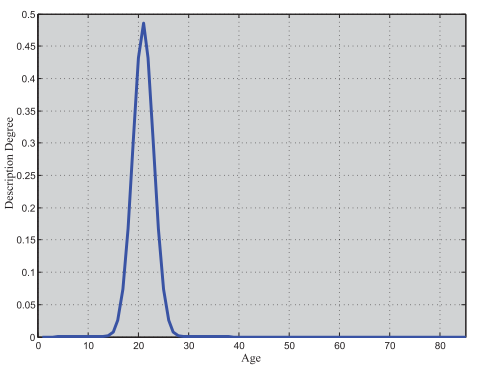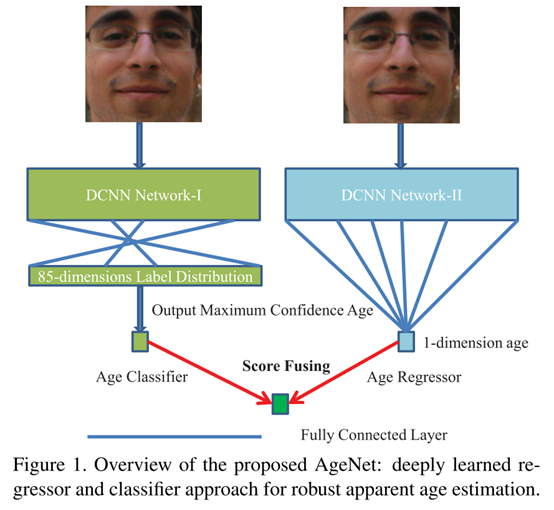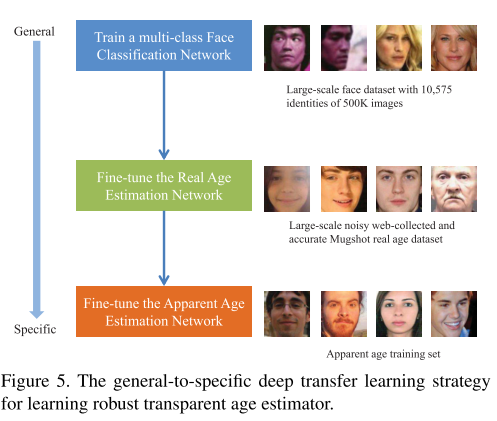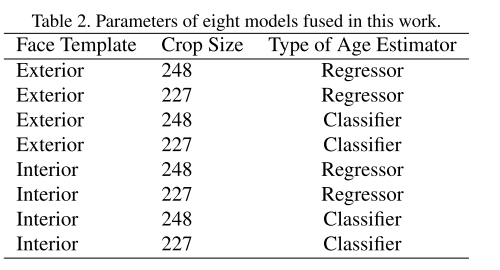0%

创新点

1. 融合了两种模型，基于实数值的回归模型以及基于高斯标签分布(Gaussian label distribution)的分类模型，两种模型都使用深度卷积神经网络来提取有表达力的年龄信息。

2. 为了避免网络在很小的表观年龄数据集上过拟合，采用了一种从通用到特定(general-to-specific)训练方式；首先使用大规模的从互联网收集人脸图像进行分类预训练，随后使用大规模的带有噪声的真实年龄数据集进行微调，最后才在很小的表观年龄训练数据集上进行微调。

基础概念1. 1-dimension real-value encoding

用一个实数值代表一个人的年龄，可以用回归模型进行建模。

2. 0/1 encoding

把不同的年龄当做不同的类，即[0,0,0…,1,0,0]，把年龄估计看做分类问题。

3. Label distribution encoding

该方法的基本思想是使用每个label中的description degree来表达每一个label实例。本文具体用的是高斯标签分布，具体表述如下：对于一张给定的图片I，如果它的年龄是y，那么使用一个多维向量来表达这个年龄label，这个多维向量的第j维是：

$$l_j=exp(\frac{-(j-y)^2}{2*\sigma^2})/\sigma,j=1,...,M$$

j代表第j维(1,2,3….85)，y是给出的年龄label，$\sigma$是label的标准差(因为label是由多个志愿者给出的，因此会有一个标准差)，M是特征向量的最大维度(同时也是训练时可能遇到的最大年龄)。下图是一个示例：网络总体框架Deeply Learned Age Regressor

$$E(W)=\frac1{2N}\sum_{i=1}^N||\hat y_n-y_n||_2^2$$

$$R=f(\hat y_n*100+0.5)$$

Deeply Learned Age Classifier

$$E(W)=\frac{-1} N \sum_{i=1}^N \sum_{n=1}^L[p_{in}log\hat p_{in}-(1-p_{in})log(1-\hat p_{in})]$$

从通用到特定的训练方法1. 不预训练直接随机初始化训练网络时效果不好，因为网络在小的训练集上面过拟合。

2. 预训练人脸多分类网络比在真实年龄数据集上预训练效果更好，意味着从与相似的任务上使用大规模数据集训练可以提升网络的泛化性。

3. 尽管互联网搜集的人脸真实年龄标签有很多噪声，但是还是可以帮助提升表观年龄估计的性能，证明了这个从通用到特殊的迁移学习方法的鲁棒性。